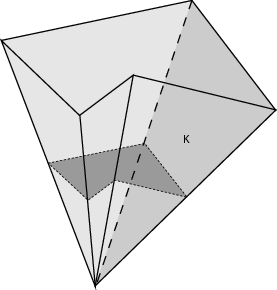# Besov regularity of parabolic partial differential equations on Lipschitz domains

## Participants

### AM3

• Cornelia Schneider

### Marburg

• S. Dahlke

Parabolic PDEs are very often used in science and engineering since they model all kinds of diffusion phenomena such as the diffusion of heat in media. A lot of results concerning existence and uniqueness of solutions are known. However, in most cases, an analytic expression of the solution is not available, so that numerical algorithms for the constructive approximation of the unknown solution up to a given tolerance are needed.
The numerical treatment of realistic problems, e.g. in 3D, leads to systems involving thousands or even millions of unknowns. Therefore, it would be tempting to use adaptive schemes in order to increase efficiency, where the degrees of freedom are adjusted step by step to the unknown solution in the sense that more degrees of freedom are spent in regions where the local error is still large.

In this project, we shall mainly be concerned with approximation schemes based on wavelets. In is known that in many cases the order of convergence of adaptive wavelet-schemes depends on the regularity of the solution in specific scales of Besov spaces. On the other hand it is the Sobolev regularity which determines the rate of convergence of non adaptive (uniform) algorithms.

In order to justify the use of adaptive schemes for solving parabolic PDEs, an analysis of the regularity of the solution in the scale of Besov spaces and a comparison with its Sobolev regularity is needed. For the case of elliptic PDEs, a lot of positive results in this direction are already known. If the domain under consideration, the right–hand side and the coefficients are sufficiently smooth, then the problem is completely regular, and there is no reason why the Besov smoothness should be higher than the Sobolev regularity. However, on general Lipschitz domains and in particular in polyhedral domains (e.g. the polyhedral cone in the figure), the situation changes dramatically. For then, singularities at the boundary may occur caused by the vertices and edges that diminish the Sobolev regularity of the solution significantly.

It is the aim of this project to investigate the corresponding situation for parabolic PDEs, which are mathematically more difficult to handle, and justify the use of adaptive schemes in this context.